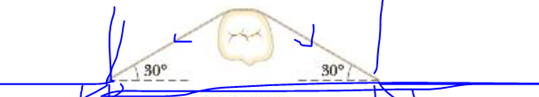Chapter 9, Problem 72P

Chapter
Section
Textbook Problem

A stainless-steel orthodontic: wire is applied to a tooth, as in Figure P9.72. The wire has an unstretched length of 3.1 cm and a radius of 0.11 mm. If the wire is stretched 0.10 mm, find the magnitude and direction of the force on the tooth. Disregard the width of the tooth and assume Young’s modulus for stainless steel is 18 × 1010 Pa.Figure P9.72

To determine
The magnitude and direction of the force on the tooth.

Explanation
The necessary tension in the wire to stretch it is T=F=[YA(ΔL)]/L0 and there are two tensional force acts on the tooth in a different direction. The horizontal components of this force would be zero and only vertical components of this force survive for the resultant force on the tooth that is R=2Fsinθ=2[Yπr2(ΔL)sinθ]/L0 .

Given info: The unstretched length of the orthodontic wire is 3.1cm , radius of the wire is 0.11m , Young’s modulus of the wire is 18×1010Pa , angle of the tensional force from horizontal is 30° , and the stretched length of the wire is 0.10mm .

The formula for the of the force on the tooth is,

R=2Yπr2(ΔL)sinθL0j^

• Y is Young’s modulus of the wire.
• r is radius of the wire.
• ΔL is stretched length of the wire.
• θ is angle of the tensional force from horizontal.
• L0 is unstretched length of the orthodontic wire.

Substitute 18×1010Pa for Y , 0

Still sussing out bartleby?

Check out a sample textbook solution.

See a sample solution

The Solution to Your Study Problems

Bartleby provides explanations to thousands of textbook problems written by our experts, many with advanced degrees!

Get Started

Find more solutions based on key concepts